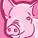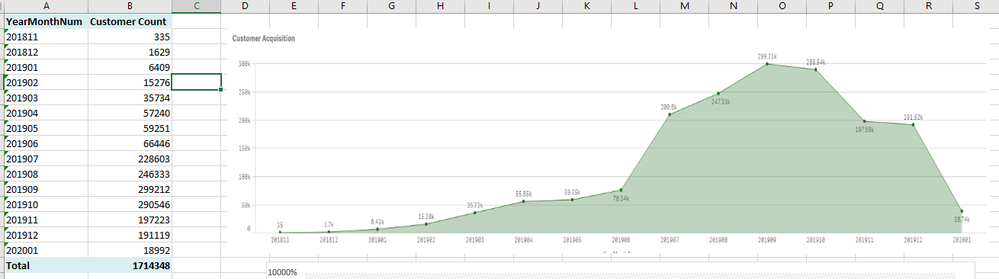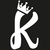# App Development

Announcements
April 22, 2PM EST: Learn about GeoOperations in Qlik Sense SaaS READ MORE
cancel
Showing results for
Did you mean:Contributor II

## Monthly Growth Rate Min to Max dates

Hi Everyone!

Can you guys help me with my formula? I have a trend chart that counts Total customer count acquisition monthly from 2018 up to latest date and I want to create another trend chart that shows its Growth Rate %.

My Original Trend chart is below:The customer count formula for that is:

COUNT({\$<DateBasis={"X"}>}  distinct [Y])

Formula that I tried:

1. ((COUNT({\$<DateBasis={"X"}>}  distinct [Y]))/(Before(COUNT({\$<DateBasis={"X"}>}  distinct [Y])) - 1) * Avg(1)))

2.  (COUNT({\$<DateBasis={"X"}, MonthNum={\$(=Max(MonthNum))}>}  distinct [Y])) -

(COUNT({\$<DateBasis={"X"}, MonthNum={\$(=Max(MonthNum)-1)}>}  distinct [Y])) /

(COUNT({\$<DateBasis={"X"}, MonthNum={\$(=Max(MonthNum)-1)}>}  distinct [Y]))

Thank you so much and have a great day!

Labels (5)

• ### trend chart

3 RepliesPartner

Hi

Try this

(Count({\$<DateBasis={"X"}>}  distinct [Y])-Below({\$<DateBasis={"X"}>}  distinct [Y]))
/
({\$<DateBasis={"X"}>}  distinct [Y])

Note : Make sure to sort by ascending order the yearmonth and give fnumber format to numer and set % format

Hope this helps

ThanksContributor II
Author

Unfortunately, it does not give me any result 😞 I just get ' - ' as a result in each row(I am trying the formula in a table first and splitting each expression into column to see where it went wrong but each doesn't gave me any result)

My dimension - ' YearMonthNum'

Measurement - 'Customer Count'(Current measurement that I want to convert into Growth Rate %) = COUNT({\$<DateBasis={"X"}>}  distinct [Y])

Regards,``````=num(alt((COUNT({\$<DateBasis={"X"}>}  distinct [Y])-